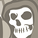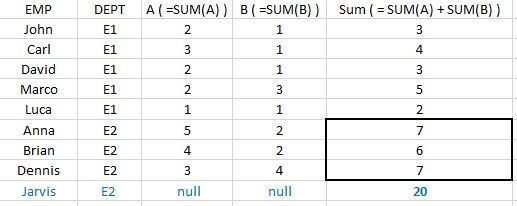Announcements
The way to achieve your own success is the willingness to help somebody else. Go for it!
cancel
Showing results for
Did you mean:Contributor II

## Displaying department results for certain employees

Hello,

I am trying to display department results only for certain employees. Please see below simplified case.

For employee Jarvis (or those, who do not have A and B values), i wish to display those sums, grouped by his DEPT.How can I do this with set analysis?

Labels (3)

• ### sums

1 Solution

Accepted SolutionsMVP

on frond end, create a straight table

Dimension:

Employee

Dept

Expression:

=if((sum(A)+Sum(B))=0,
sum(total <Dept> aggr(sum(A)+Sum(B),Dept)),sum(A)+Sum(B))

2 RepliesMVP

Would you need script solution or front end?MVP

on frond end, create a straight table

Dimension:

Employee

Dept

Expression:

=if((sum(A)+Sum(B))=0,
sum(total <Dept> aggr(sum(A)+Sum(B),Dept)),sum(A)+Sum(B))Tags
Community Browser